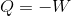# High School Physics : Understanding the First Law of Thermodynamics

## Example Questions

### Example Question #4 : Thermodynamics

A gas in a closed container is heated with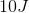of energy, causing the lid of the container to rise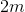with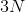of force. What is the total change in energy of the system?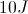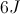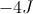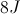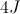Explanation:

For this problem, use the first law of thermodynamics. The change in energy equals the increase in heat energy minus the work done.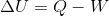We are not given a value for work, but we can solve for it using the force and distance. Work is the product of force and displacement.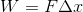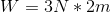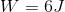Now that we have the value of work done and the value for heat added, we can solve for the total change in energy.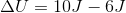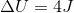### Example Question #5 : Thermodynamics

A gas in a closed container is heated, causing the lid of the container to rise. The gas performsof work to raise the lid, such that is has a final total energy of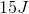. How much heat energy was added to the system?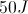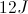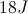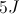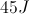Explanation:

For this problem, use the first law of thermodynamics. The change in energy equals the increase in heat energy minus the work done.We are given the amount of work done by the gas and the total energy of the system. Using these values, we can solve for the heat added.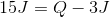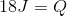### Example Question #6 : Thermodynamics

A gas in a closed container is heated with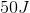of energy, causing the lid of the container to rise. If the change in energy of the system is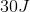, how much work was done by the system?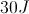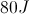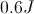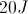Explanation:

For this problem, use the first law of thermodynamics. The change in energy equals the increase in heat energy minus the work done.We are given the total change in energy and the original amount of heat added. Using these values, we can solve for the work done by the system.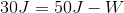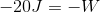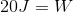### Example Question #7 : Thermodynamics

Which of the following represents the first law of thermodynamics?

If a refrigerator is unplugged, eventually everything inside of it will return to room temperature

While melting, an ice cube remains at the same temperature

Two systems in equilibrium with a third system are in equilibrium with each other

The specific heat of an object explains how easily it changes temperatures

After falling down the hill, a ball's kinetic energy plus heat energy equals the initial potential energy

After falling down the hill, a ball's kinetic energy plus heat energy equals the initial potential energy

Explanation:

The first law of thermodynamics is another wording of the law of conservation of energy. Effectively it states that energy cannot be created or destroyed, but it can change forms.

This means that, in the given situation of the ball rolling down the hill, the total initial energy equals the final kinetic energy plus heat.

The zeroth law of thermodynamics states that if a system is in equilibrium with two other systems, then the two other systems are in equilibrium with each other.

The second law of thermodynamics states that the entropy of a closed system will always increase.

The third law of thermodynamics states that absolute zero is the temperature at which entropy is zero.

### Example Question #8 : Thermodynamics

According to the first law of thermodynamics, in an isothermal process __________.

temperature is not affected by the pressure or volume changes of the system

a constant amount of heat is added to the system

temperature is not affected by the internal energy of the system, work, or heat

it can be implied that heat added to the system is equal to work done by the system

internal energy of the system fluctuates and there exists an imbalance between work and heat

it can be implied that heat added to the system is equal to work done by the system

Explanation:

According to the first law of thermodynamics, change in internal energy of a closed system is given by the difference between the heat energy added to the system and the work done by the system: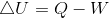In an isothermal process, temperature is constant. Temperature is a measure of internal energy of the system. If temperature is constant, then there is no fluctuation of internal energy.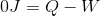It can be implied that heat added to the system is equal to work done by the system.